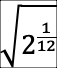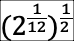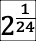## ACOUSTICS practice for midterm

1) If the frequency (f) of a sine wave is 1000 Hz, what is the period (T) of that wave?

T = 1/f so T = 1 msec

2) If the period (T) of a wave is 10 msecs, what is its frequency?

f = 1/T so f = 100 Hz

3) If the period (T) of a wave is 100 msecs, what is its frequency?

f = 1/T so f = 10 Hz

4) If the frequency (f) of a sine wave is 5000 Hz, what is the period (T) of that wave?

T = 1/f so T = .2 msec

5) If the frequency (f) of a sine wave is 110 Hz, what is the period (T) of that wave?

T = 1/f so T = 1/110 = 9.1 msec

6) A sound has an amplitude of 60 dB. What would be the resultant dB of the sound if:

1. the intensity is halved -- 57 dB
2. the pressure is doubled -- 66 dB
3. the intensity is multiplied by 10 -- 70 dB
4. the pressure is divided by 3 -- 60 - 9.5 = 50.5 dB

7)    Two tones of 440 Hz and 444 Hz are played.  The beat frequency is:

a)  440 Hz
b)  444 Hz
c)  442 Hz
d)  4 Hz

8)    Which of the following will not affect the fundamental frequency of vibration of a string?

a)  changing the amplitude of vibration
b)  changing the tension of the string
c)  changing the length of the string
d)  changing the density of the string

9)    Compared to the velocity of a 400 Hz sound through air, the velocity of a 200 Hz sound through air is

a)  twice as great
b)  one-half as great
c)  the same

10)   If the displacement of vibrating particles is perpendicular to the direction of
propagation of the wave, the wave is said to be:

a)  linear
b)  transverse
c)  longitudinal

11)    There are two organ pipes of the same length.  One has one end closed, while the
other has both ends open.  The one with a closed end will emit sound of

a)  a higher frequency
b)  a lower frequency (to be precise -- an octave lower)
c)  the same frequency

23)    When a stretched wire (fixed at both ends) is vibrating in the second harmonic, there
is/are

a)  one node
b)  two nodes
c)  three nodes

12)    Two pure tones cause resonance in different positions along the basilar membrane.
These tones have different:

a)  amplitude
b)  frequency
c)  timbre

13)    Harmonics have wavelengths, which compared to the fundamental are:

a)  longer
b)  shorter
c)  the same

14)    A sound pressure level of 110 decibels would be considered

a)  very loud
b)  average for speaking
c)  very soft

15)

 RATIO dB intensity dB pressure 1:6000 1:1000 1:2 (one half) -3 dB -6 dB 1:1 2:1 3 dB 6 dB 3:1 4.8 dB 9.6 dB 5:1 6:1 10:1 10 dB 20 dB 20:1 100:1 20 dB 40 dB 200:1 1,000:1 6,000:1 20,000:1

 RATIO dB intensity dB pressure 1:6000 -37.8 -75.6 1:1000 -30 -60 1:2 (one half) -3 dB -6 dB 1:1 0 0 2:1 3 dB 6 dB 3:1 4.8 dB 9.6 dB 5:1 7 14 6:1 7.8 15.6 10:1 10 dB 20 dB 20:1 13 26 100:1 20 dB 40 dB 200:1 23 46 1,000:1 30 60 6,000:1 37.8 75.6 20,000:1 43 86

16) What frequency is a quartertone (half of an equal-tempered semitone) sharp of A440?

A quartertone is half of an ETST (equal-tempered semitone), so it's==To go up by this amount, multiply: 440 *= 452.9 Hz

17) How many cents is the pythagorean comma?

just plug in the values:== 23.46 cents

18) How many cents in the equal tempered tritone? (C to F# on the piano)

100 cents per ETST, so 100 * 6 = 600 cents

19) Show that in just intonation there are two different whole-steps.

To do this, you need to have all the ratios for just intonation; then you simply find all the major 2nds (whole steps) in the scale (there are 5 of them). For example, the first interval (from C to D) is 9/8. The next interval (from D to E) is 5/4 ÷ 9/8 = 5/4 * 8/9 = 10/9. Do this calculation also for F to G, G to A and A to B.

20) Four trombones are playing together, each at 80 dB. What is the intensity of the resulting sound?

For uncorrelated signals, the increase is +3 dB per doubling, so the answer is 86 dB